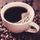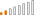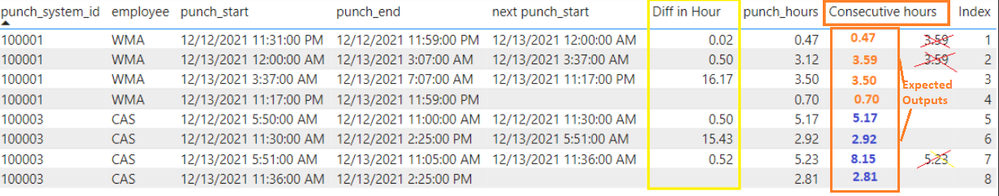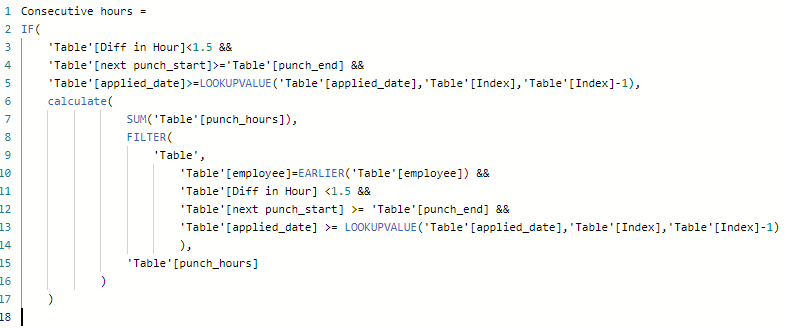cancel
Showing results for
Did you mean:Continued Contributor

## Calculate sum of Consecutive hours with condition

I am sharing my Pbix file here.

I am trying to calculate "Consecutive Hours" based on this condition:If employee works continously without having more than 1.5 hours as interval (Diff in Hour) between each shift, it will add hours together as Consecutive hours.

For example, employee WMA worked 0.47 hours, and then on next shift, WMA works 3.12 hours with interval of 0.5 hours (which is less than 1.5 hours), so we add 0.47 + 3.12 = 3.59 (Expected output).

But, on the next shift, WMA's interval was 16.17 hours (which is more than 1.5 hours), punch_hours of 3.50 does not get added up to previous sum of 3.59. So, the output should be 3.50 (Expected output).

Same scenario for employee CAS:

CAS worked 5.17 hours, but on the next shift, interval is 15.43 hours (>1.5 hours), so 2.92 (expected output) does not get added upto 5.17.

But on the next shift, interval is only 0.52 hour, 2.92 hour gets added upto 5.23 hours (8.15 = 5.17 + 2.92).

Here the expected output is 8.15.

Diff in Hours basically calculates difference between punch_end and next punch_start.

Diff in Hour = (DATEDIFF('Table'[punch_end],'Table'[next punch_start],MINUTE)/60)

I am trying to fix the measrue for Consecutive Hours.

This is what I have:

Consecutive hours =
IF(
'Table'[Diff in Hour]<1.5 &&
'Table'[next punch_start]>='Table'[punch_end] &&
'Table'[applied_date]>=LOOKUPVALUE('Table'[applied_date],'Table'[Index],'Table'[Index]-1),
calculate(
SUM('Table'[punch_hours]),
FILTER(
'Table',
'Table'[employee]=EARLIER('Table'[employee]) &&
'Table'[Diff in Hour] <1.5 &&
'Table'[next punch_start] >= 'Table'[punch_end] &&
'Table'[applied_date] >= LOOKUPVALUE('Table'[applied_date],'Table'[Index],'Table'[Index]-1)
),
'Table'[punch_hours]
)
)I am not sure where I need to fix.

1 ACCEPTED SOLUTIONNew Member

The identical question was posted a few days ago. The community provided some suggestions: https://community.powerbi.com/t5/DAX-Commands-and-Tips/SUMX-Calculation/m-p/2787583#M87425New Member

The identical question was posted a few days ago. The community provided some suggestions: https://community.powerbi.com/t5/DAX-Commands-and-Tips/SUMX-Calculation/m-p/2787583#M87425